# Intuitive Deep Learning Part 3: RNNs for Natural Language Processing

Source: Deep Learning on Medium

Where we left off: In part 1, we had an introduction to neural networks and how to make them work. We started off in Part 1a with a high-level summary of what Machine Learning aims to do:

Machine Learning specifies a template and finds the best parameters for that template instead of hard-coding the parameters ourselves. A neural network is simply a complicated ‘template’ we specify which turns out to have the flexibility to model many complicated relationships between input and output. Specifying a loss function and performing gradient descent helps find the parameters that give the best predictions for our training set.

In part 2, we looked at how we can apply neural networks to images using CNNs:

Images are 3-dimensional array of features: each pixel in the 2-D space contain three numbers from 0–255 (inclusive) corresponding to the Red, Green and Blue channels. Often, image data contains a lot of input features. A layer common in CNNs in the Conv layer, which is defined by the filter size, stride, depth and padding. The Conv layer uses the same parameters and apply the same neuron(s) across different regions of the image, thereby reducing the number of parameters needed. Another common layer in CNNs is the max-pooling layer, defined by the filter size and stride, which reduces the spatial size by taking the maximum of the numbers within its filter. We also typically use our traditional Fully-Connected layers at the end of our CNNs. AlexNet was a CNN which revolutionized the field of Deep Learning, and is built from conv layers, max-pooling layers and FC layers. When many layers are put together, the earlier layers learn low-level features and combine them in later layers for more complex representations.

For our neural networks thus far to work, however, notice that we take in an input of fixed length / size and we give an output of fixed size as well. The input size and output size specifies the number of parameters that we need, and so we need that fixed well in advance before we can do any model training. When we get to applications on Natural Language Processing and other sequence data, we encounter the problem: Sentences have varying length; how do we apply neural networks to sentences?

Before we start our introduction to RNNs, it is important to understand how words are understood by the machine. After all, our neuron takes in numbers as features rather than words. How would our neuron (and ML algorithms in general) understand words then?

Firstly, let’s break down the problem. There are way too many words in an English dictionary, add in non-dictionary words (such as names) and things get complicated. So what we can do is take the most common 30,000 words and say that our computer can only understand these 30,000 words. We call this our vocabulary. Any word outside this 30,000 words will be understood as “Unknown”, or “UNK”. Note that UNK is also a word we will add to our vocabulary.

Once we’ve found our vocabulary, this is one approach in which we can convert words into numbers. Let’s use an example to make things clear. Suppose we’ve built our vocabulary of 30,000 words as below:

Each word corresponds to an index, which is the position of that word in the vocabulary. Suppose we wish to find a representation for the word ‘hello’, which is the 13,941-th word in our vocabulary.

What we can do is to represent the word as a set of 30,000 numbers (x1, x2, … x30000). We call this set a vector. What do we set the numbers to for the word ‘hello’? We set x13941 = 1 since ‘hello’ has index 13,941 in our vocabulary. All the other features (x1, x2, …, x13940, x13942, …, x30000) will be set to 0.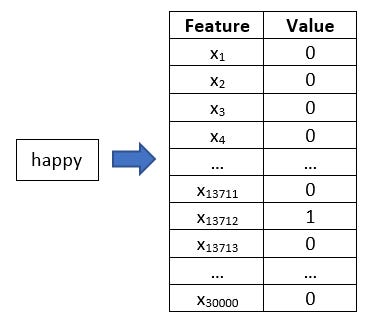The feature x13941 will have the value 1 since ‘hello’ is in the 13,941-th position of our vocabulary. All other features will take on the value 0.

Intuitively, you can think of this method as a ‘count’ feature. We take an input word (in our example, ‘hello’) and count how many times we see the respective words in our vocabulary? So we start from ‘a’, our first word in the vocabulary, corresponding to the feature x1. How many times do we see the word ‘a’ in the input sentence ‘hello’? None, so we set the value of x1 to be 0. We continue until we reach x13941. How many times do we see the word ‘hello’ (the 13,941-th word in our vocabulary) in the input sequence ‘hello’? We see it once, so we set x13941 = 1. And we continue down our vocabulary likewise.

This method of changing a word into a vector with ‘1’ for the feature corresponding to that word and ‘0’ otherwise is called one-hot encoding.

Now we’ve got a method to convert words into numbers. But is this the best way? Suppose we have two words, ‘happy’ and ‘joyful’. These two words will be represented as the following: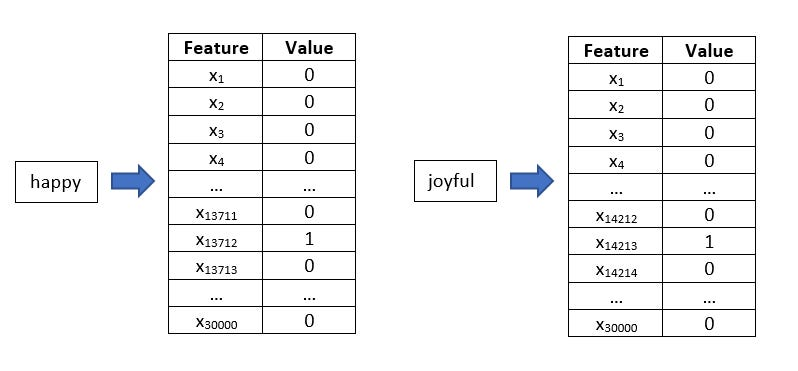The one-hot encodings of the word ‘happy’ (in the 13,712-th position) and the word ‘joyful’ (in the 14,213-th position)

Note that nowhere within our representation do we know that ‘happy’ and ‘joyful’ are actually synonyms and have similar meaning! Words represent meaning and are not just arbitrary sequences of characters, but this has not been represented in our method. In other words, the one-hot encoding was successful in converting our words into a set of numbers, but the numbers do not encode the meaning behind the word.

A popular method that addresses this issue is called word2vec. The exact details of how word2vec is created is found in Footnote 1, but if you’re not interested in that, just take it that people out there have created this magical function for you to convert a word into a set of 300 numbers (well, the size of the vector can vary — but 300 is the most common). These 300 numbers encode some of the meaning of the word, so words like ‘happy’ and ‘joyful’ will be similar to each other since they have similar meanings.

One interesting property about word2vec is that you can actually ‘subtract’ or ‘add’ word meanings from other word meanings. After converting all the words into the vector of 300 numbers, one interesting experiment to ask is: What if I subtract or add the vectors that represent those words?

For example, what if I do the operation on the vectors that represent the words as such: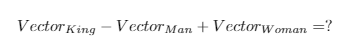We take the vector representing the word ‘king’, subtract it by the vector representing the word ‘man’ and add the vector representing the word ‘woman’. What should we get?

What researchers found was that the above resulted in the vector corresponding to the word ‘Queen’. As compared to our one-hot encoding, these word2vec vectors have encoded information behind what the word means, including attributes such as gender.

An alternate way to think of encoding meaning is to extract features ourselves. For example, we can postulate that a meaning behind a word is defined by its gender (male = 1, female = -1), its ‘royalty’ (royal = 1, not royal = -1) and whether it is a person (person = 1, not person = -1) amongst other things. We could think of the vector addition / subtraction like this: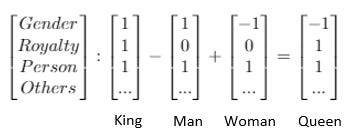If we encoded meaning using the attributes of ‘gender’, ‘royalty’ and ‘person’ amongst others, this could be what was happening with the vector operations.

However, in Deep Learning, what each number represents is not human-interpretable like in our example above. The machine just figured out the best way to represent those numbers in a non-human-interpretable manner, such that it encodes the meaning of the word well.

There are many ways of encoding meaning, and in this post we only discuss one of such methods; however, the intuition and purpose behind finding a vector to represent the meaning of the word remains similar.

Summary: In word2vec, words are converted to a vector of 300 numbers which attempts to encode the meaning behind the word.

Now that we know how to convert words into numbers to feed in to the machine, the question remains: how do we deal with sentences where there are a variable number of words?

One solution to this problem is to specify a maximum number of words that a sentence can have. Suppose that number is 90. If our actual sentence has 10 words, then we fill all the other 80 spots with a special token called <pad>. This technique is called padding, and you might have seen something similar with CNNs. After padding our inputs, we will always have a fixed-size sequence of words, and so here we can use our traditional algorithms (e.g. CNNs).

Another solution, which we introduce here, is using Recurrent Neural Networks, or RNNs. In RNNs, we don’t take the input all at once to predict some output. To illustrate the use of RNNs, we will tackle the problem of translating the sentence “I am a cat” into French. This is a challenging problem because not only are the inputs (English sentence) variable length, the outputs (French sentence) are often variable lengths as well.

There are many ways we can arrange RNNs depending on the problem, giving rise to a variety of RNN architectures. We won’t go through all of them, but we will go through one of them in depth to get an intuition of RNNs. For this problem of translating from English to French, we will use the encoder-decoder architecture. As the name suggests, we break down the problem into two bits:

1. Encoder: Encode the sentence “I am a cat” into some set of numbers (vector). You can think of this set of numbers as representing the meaning of “I am a cat”.
2. Decoder: Decode this set of numbers into the respective French sentence, which is “Je suis un chat”.

Summary: We can use the encoder-decoder RNN architecture for the problem of machine translation.

We introduce another vector that we call the ‘hidden vector’. For now, think of it as the ‘sentence meaning vector’, although this terminology is technically inaccurate. But for the sake of getting some intuition, we’ll work with this for now. The job of the RNN is then to learn how to encode new words into the hidden vector (sentence meaning vector), rather than to map the input to output directly.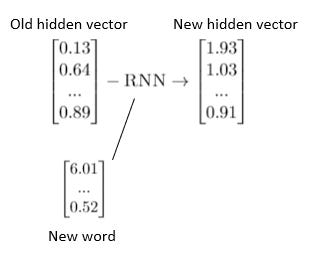The job of the RNN is to figure out how to take the old hidden vector (sentence meaning vector) and combine it with the new word to give a new hidden vector (sentence meaning vector)

The RNN processes the features by concatenating the two vectors together. Remember that each number in the hidden vector is simply a feature, and each number in the word vector representing the new word is also simply a feature. If our hidden vector had 1000 features and our new word vector had 300 features, then the RNN takes in as input 1300 features. And through our RNN, learn how these features can ‘combine’ together.

By using this RNN that takes the old hidden vector and a new word vector to output a new hidden vector, we can read the English sentence word-by-word to encode the full sentence “I am a cat” into the final hidden vector. For clarity purposes, we go through a step-by-step run down:

The hidden vector starts off with all zeros since there is no meaning at the start. We call this h0. Then, we look at the first word, “I”. Our neural network take the old hidden vector (h0) and combine it with the word vector that represents “I” (e.g. word2vec). Now, our hidden vector h1 is a set of numbers that represents the sentence “I”.

Then, we look at the second word, “am”. We use the exact same neural network that takes the hidden vector h1 and combine it with the word “am” to produce a hidden vector h2 that represents the sentence “I am”. This is another form of parameter sharing: we use the same function to encode each word, but the inputs are different at each step. We call each step here a time-step.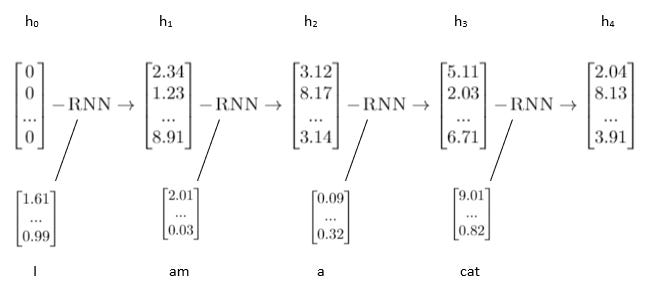An example of how a RNN encodes into the hidden vector new words. Note that the RNN used throughout is the same function, but different inputs are given at each time step.

We continue on. We look at the third word, “a”, and use the exact same neural network to combine the hidden vector h2 with the word “a” to produce a hidden vector h3 that represents the sentence “I am a”.

Lastly, we look at the fourth word “cat”, and use the exact same neural network to combine the hidden vector h3 with the word “cat” to produce a hidden vector h4 that represents the sentence “I am a cat”. The figure for encoding the words in order is shown below:

Now that we have a vector representing “I am a cat”, we’ll use this vector to convert it into French words.

But before we do so, let’s just highlight again the RNN approach. With RNNs, we deal with the problem of varying inputs not by padding but by changing the problem definition of what our neural network does. A traditional neural network might see the problem as:

Input: Sentence of input English words; Output: Sentence of French words.

A RNN (at the encoding step) instead sees this problem as:

Input: Previous hidden vector and new word vector; Output: New hidden vector that combines the meaning of the old hidden vector and this new word.

Note that since the previous hidden vector and the new word vector has a fixed input size, our neural network can deal with the inputs normally. However, since our neural network can keep encoding new words, there is no technical limit to the length of the sentence it inputs.

When it reaches the end of the sentence, there is a special word (or token) that is given to the neural network. This token is called the End-of-Sentence token, and is commonly represented by <EOS>. Once the neural network sees this, it knows that we’re done with encoding new words and moves on to the decoder part of the neural network.

Summary: The RNN in the encoder learns how to combine previous hidden vectors with new words it encounters to form new hidden vectors. The hidden vector at the end of the sentence represents the meaning of the sentence it just saw.

We now move on to the decoder.

Now that we have a hidden vector representing “I am a cat”, it is time to convert this hidden vector into a French sequence of words with our decoder! This time, we use another RNN, which is different from our encoder.

Two things are happening here at each decoder step:

1. Output the French word from the hidden vector,
2. Remove that word from the hidden vector so that the new hidden vector represents the words ‘to be translated’.

Step 1: Output the French word from the hidden vector. For this, we have another layer which takes the hidden vector at that step and transforms it to a probability distribution over the words in the vocabulary (using softmax).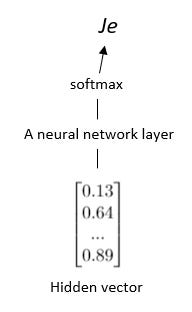We pass the hidden vector through a neural network layer, apply softmax to get a probability distribution over all the French words in the French vocabulary. In this case, “Je” is the most probable word.

Note that the neural network layer to transform the hidden vector into the probability distribution over the French vocabulary is the same at all steps. In this case, our neural network is learning to translate hidden vectors into French words.

Step 2: We are able to output one word, but how do we output multiple words? Having one output might have been sufficient for predicting sentiment, but not for translating full sentences.

Our RNN here at Step 2 of our decoder is attempting a very similar problem as the RNN in our encoder: given the previous hidden vector and the previous word seen, output the next hidden vector.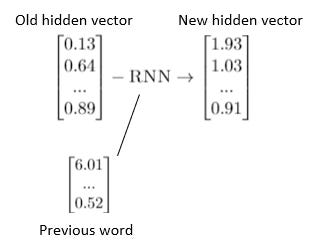The RNN for the decoder takes the previous word and the old hidden vector to output a new hidden vector

The intuition, however, is very different. What we are trying to do is ‘subtract’ the meaning of the previous word from the hidden vector. The resulting hidden vector then represents the words yet to be translated. When there is nothing left to be translated, the word we will get from the hidden output at that point is simply the End-of-Sentence token (<EOS>), telling us that our job is done and we need not continue on the decoding sequence.

You might now be wondering at this stage: How do we get the previous word seen? From the output of Step 1! This is how Step 1 and Step 2 comes together: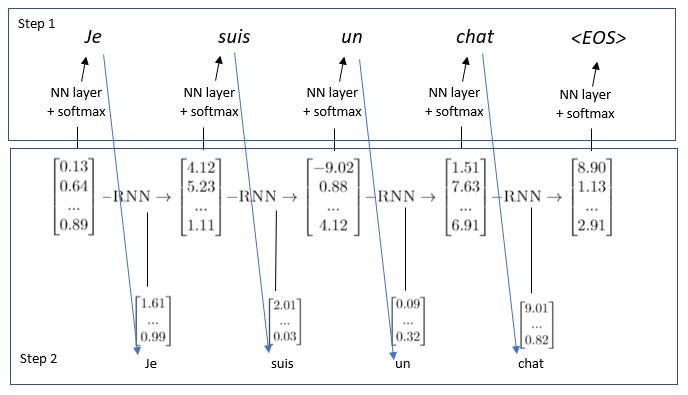How the two steps come together. The output of the previous word (from Step 1) becomes an input in the RNN in Step 2. This continues until the token is predicted and the decoding stops.

This might be the most complicated diagram you’ll see in this series of posts, but it outlines the overall decoding architecture. Similar to the encoder, we make the distinction between a traditional neural network and our RNN. A traditional neural network might see the problem as:

Input: Sentence of input English words; Output: Sentence of French words.

A RNN (at the decoding step) instead sees this problem as:

Input: Previous hidden vector and previous word predicted; Output: New hidden vector that subtracts the word out from the ‘words yet to be translated’.

It’s not the most intuitive concept, but I think that it is an ingenious way of dealing with variable-sized inputs and outputs. And for the most part, it works well! There are a few issues with the vanilla RNN that we’ve yet to talk about, and this will require us to tweak our RNN a little more before it’s ready. In the more advanced posts, we will talk about those problems and the tweaks such as LSTM and Attention.

Summary: The decoder does two things to unwrap the hidden vector into the sequence of French words. Firstly, we output a probability distribution of the French word at that time-step. Secondly, we produce a new hidden vector that represents the words left to be translated. This continues until we predict the <EOS> token.

Consolidated Summary: In word2vec, words are converted to a vector of 300 numbers which attempts to encode the meaning behind the word. We can use the encoder-decoder RNN architecture for the problem of machine translation. The RNN in the encoder learns how to combine previous hidden vectors with new words it encounters to form new hidden vectors. The hidden vector at the end of the sentence represents the meaning of the sentence it just saw. The decoder does two things to unwrap the hidden vector into the sequence of French words. Firstly, we output a probability distribution of the French word at that time-step. Secondly, we produce a new hidden vector that represents the words left to be translated. This continues until we predict the <EOS> token.

What’s Next: Now that we have looked at how neural networks are applied to text, we will shift our focus to using neural networks for reinforcement learning in Part 4 (link). Reinforcement learning is behind many of the achievements you might have heard of in the news, such as AlphaGo and AlphaZero beating the top Go players, or DeepMind’s achievement of learning to play Atari games from merely the pixel data alone.

Footnotes:

1. word2vec

word2vec is actually not a single model, but a software package that contains a group of models which convert words to vectors. One of those models is called the skip-gram model.

The intuition behind the model is that you can tell the meaning of a word by the words surrounding it. Let’s consider this examples:

I enjoy drinking _______ juice.

What can you tell about the blank word from its context? It’s likely to be a fruit, since there is the word ‘juice’ in it. Its likely to be an edible fruit, since we would not want to drink juice from fruits that are poisonous. It’s likely to be a fruit whose juice people enjoy drinking too.

Arguably, words that fit the above descriptions such as ‘apple’ or ‘orange’ should thus be close together in the representation since they represent all the meaning as above. If we give many different training examples (i.e. many different contexts), we can get a pretty holistic view of the meaning similarity between two words.

We use this insight to generate our skip-gram model. The set-up is as follows:

Look at the word-pairs within a certain window. Let’s say our window is two words to the left and right. If we take the sentence, “I enjoy drinking apple juice”, the word-pairs with the word apple would be: (apple, enjoy) (apple, drinking) and (apple, juice).

Our model then tries to predict what are likely surrounding words. So if we input to the model the word “apple”, the output should give a relatively high probability to the words “enjoy”, “drinking” and “juice”.

The architecture of our model is as follows:

• Input layer: One-hot encoding of the word. The size of a one-hot vector is the size of the vocabulary, V
• Hidden layer: 300 neurons
• Output layer: Probability distribution over the words in the vocabulary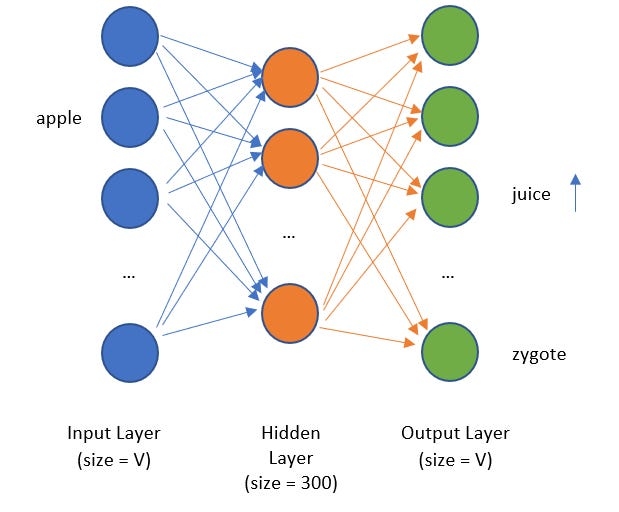The architecture of our word2vec model. A word such as ‘apple’ should predict a higher probability on words such as ‘juice’ as compared to ‘zygote’ since it is more likely for ‘apple’ to be seen together with the word ‘juice’.

What do you think the output distribution of the word ‘apple’ and ‘orange’ should look like? They should look pretty similar, since they are likely to predict surrounding words such as ‘juice’ rather than ‘zygote’. If they have similar distributions in the output layer, then they are likely to have similar hidden layer output too. This is our key insight!

By now, you might think it is too much of a coincidence that we specified 300 hidden layer neurons in our architecture above. Since words that have similar meaning will have similar hidden layers (as argued above), we can take the hidden layer as our word vector!

Predicting surrounding words is actually not the main purpose of running the task. The main purpose is to run the model and then extract out the hidden layers to act as our word2vec vectors! Cool, huh?